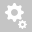##Recently viewed tickets# Composite Section for Construction Stage Stiffness Properties

Question:

Hello,

I am using the Composite Section for Construction Stage. When I check the composite stiffness values from this tab I notice that the Ixx (torsion) is not correctly calculated for the composite section. I compare this to the value directly from Section Data results. See screen shots below.

This is quite a difference and affects results a lot. Can you please explain the difference as I believe the correct value is the Section Data but this is not what the analysis uses.Figure above from Composite Section for Construction StageFigure above from Section Data

Thanks

Hello,
Greetings.

Sorry for the confusion.
The screenshot was taken from the online help manual. refer the online manual shown below which has question 1.Question 2: Secondly since there is this limitation how does it apply to the notional size of member 'h'. The values of h suggest they are for each part 1 and 2 independently. How does the software then calculate 'h' of the composite section? Before composite the girder part 1 has entire perimeter exposed however once composite part 1 and 2 combined there is now an internal void which changes the calculation for perimeter and hence 'h'.
Answer: Yes, the notional size is calculated/entered as part wise independently.
As the different parts of the composite has different age, therefore, the separate notional size for each part is calculated to obtain creep and shrinkage.
Further, the general practice is that, for part 1, h is manually calculated and entered as per enclosed section as the for most of it's service life it remains enclosed.
The midas will auto-calculate the h value of each section independently without considering it enclosed section. So, for enclosed section part, we need to update the value of h manually.

Two things to clarify.
The first is when your say this method is also described in Q1, can you please elaborate on what is Q1?

Secondly since there is this limitation how does it apply to the notional size of member 'h'. The values of h suggest they are for each part 1 and 2 independently. How does the software then calculate 'h' of the composite section? Before composite the girder part 1 has entire perimeter exposed however once composite part 1 and 2 combined there is now an internal void which changes the calculation for perimeter and hence 'h'.Hello,
Greetings.

Thanks for reaching us.
I understand the concern.
Actually, currently it is limitation of the software.So, workaround for this is giving the section stiffness scale factor as shown below. Enter the appropriate section stiffness scale factor for Ixx only.Hope this helps and kindly let me know if further assistance is required.
Creation date: 6/8/2021 2:12 PM (jatin2010)      Updated: 6/8/2021 2:12 PM ()